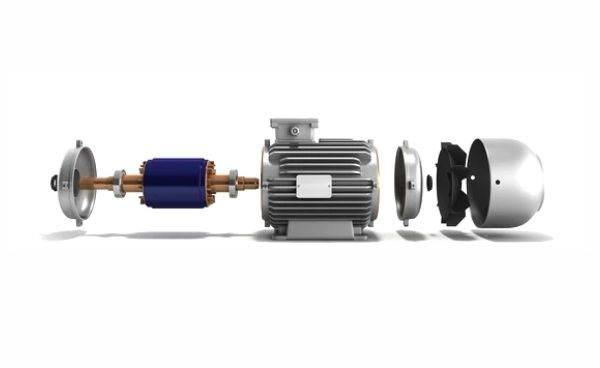# Importance of air gap for motor designThe air gap refers to an actual physical gap in an electric motor that separates the moving rotor and the stator core. air gap is a non-magnetic part of a magnetic circuit. It’s a important part of any rotating machine design.

## Importance of air gap for motor design

Air gap is a necessary part of motor design and the size of the air gap is one of the keys to motor performance and reliability.

A minimum air gap of 0.2 mm is maintained for smooth rotation of Machines. the optimum value of the air gap thickness is 0.6 mm. At this point, the motor efficiency can still reach 90%.

The length of the air-gap affects the following performance parameters of the induction motor.

1. Magnetizing current
2. Power factor
4. Cooling
5. Noise

### 1. Magnetizing Current

it is a current that magnetizes or energizes a magnetic core;

The magnetizing current of the motor depends on the specific magnetic loading and the air gap length of the machine.

A large air-gap length leads to higher magnetizing current and poor power factor.

A large air-gap length increases the reluctance of the path of the magnetic flux.

The reluctance of the magnetic circuit is similar to the resistance of the electric circuit.

```R = MMF / Φ.............(1)

Also,

R =  L / μA    .........(2)

MMF = RΦ

Φ = MMF / R

Φ = MMF * (μA /L) .....(3)

Where,
R       = Reluctance of the magnetic circuit
MMF = Magnetomotive force(MMF=NI)
L       = length of the air gap
Φ      = Flux in the air gap
μ       = Permeability of the magnetic materia```

From equation (3),

it is clear that the MMF required for producing and sending the flux through the air gap depends on the flux density and the air-gap length.

### 2. Overload Capacity of Electric Motor

The leakage flux is reduced with an increase in air gap length.

The flux produced in the stator winding gets almost fully coupled with the rotor winding if the air gap length is more.

Therefore, the overload capacity of a large air gap length motor is more than the overload capacity of the motor that has a small air gap length.

With an increase in the air gap length, the leakage reactance decrease and the overload capacity increase.

### 3. Cooling of Electric Motor

With large air gap length, the stator and the rotor are separated by large distance so cooling is better.

The copper loss (I^2*R Loss) takes place in the stator and rotor winding and, the iron loss takes place in the core and heat is easily transferred if the motor has large air gap.

The insulation of the winding can be used of H class or F class with temperature rise limit to B class if the air gap length is more.

### 4. Noise Of Electric motor

The leakage flux gets reduced with large air gap length. The less noise is generated in the motor that has more air gap length.

### What should be the optimum air gap length?

Unlike in a transformer,

it is impossible to have zero air gap for rotating machine.

```The rotating machine must have the air gap for its rotation.
The optimum air gap of an induction motor can be expressed by the following
empirical formula.

lg = 0.2 + 2√ LD    mm

Where,

lg = Air gap Length (mm)
L = Stator core Length(Meter)
D = Internal diameter of the Stator core(Meter)```
```Example:

If the stator  core length and diameter is 0.18 meter and 0.34 meter respectively,
the air gap length between the stator and the rotor

lg = 0.2 + 2√ LD    mm
= 0.2 +2√0.18*0.34
= 0.2 +0.494
= 0.694 mm```

The designer keeps the minimum air gap length in the energy-efficient motors to improve the power factor and to reduce the no-load losses in the motor

### Generally an air gap in a DC machine more than an AC motor,

In case AC motors,

The air gap should be less because the mmf required would be more otherwise and this causes the motor to draw lot of reactive power which is not desirable since the power factor becomes less and hence the torque.

Also the transmission lines capacity should be increased in order to send lot of active and reactive power.

In case of DC machines or Dc Motor,

Armature reaction distorts the flux and also the flux density on some places increases. So by keeping the air gap more we decrease the effects of armature reaction on the stator.

### Testing for Air Gap Issues

There are three methods that can be used to reveal the presence of an air gap,

• MCA (Motor Circuit Analysis)
• CSA (Current Circuit Analysis)
• RIC (Rotor Influence Check)

While they can point to an air gap, they cannot always reveal if it is getting worse over time and how much eccentricity is actually present.

### Conclusion

Electric motor air gaps can have a tremendous effect on motor performance, whether the gap is too big or too small even more complications arise when the air gap is eccentric.

Air gap size and uniformity is bigger issue with large motors and large motor repair.